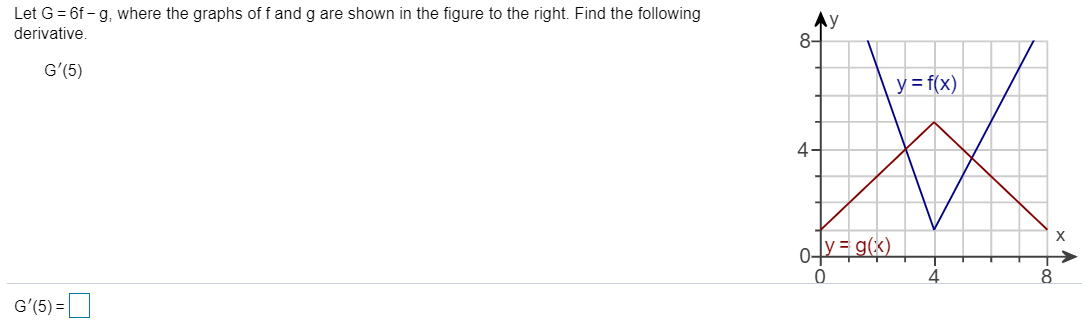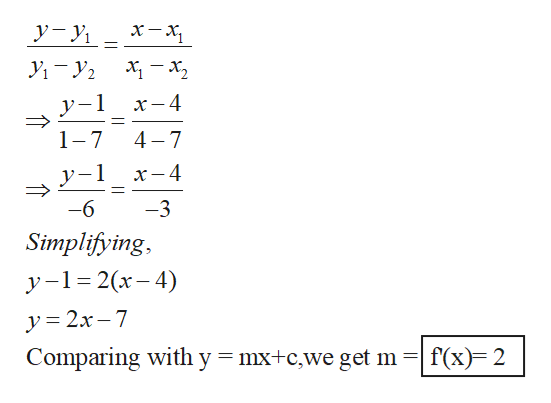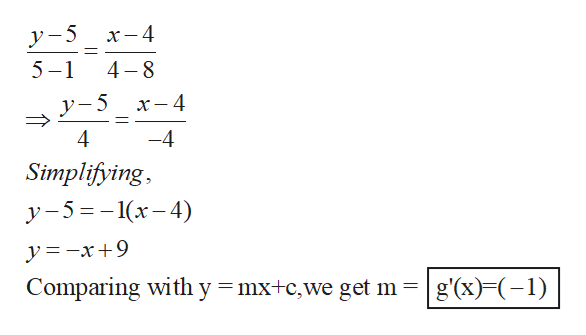# Let G 6f- g, where the graphs of f and g are shown in the figure to the right. Find the followingderivative.Ay8-G'(5)y = f(x)4-X0fygk)4G'(5)

Questionhelp_outlineImage TranscriptioncloseLet G 6f- g, where the graphs of f and g are shown in the figure to the right. Find the following derivative. Ay 8- G'(5) y = f(x) 4- X 0fygk) 4 G'(5) fullscreen
check_circle

Step 1

The given graph has two functions f(x) and g(x). We consider the interval from 4<x<8, Calculate the slopes for both the graphs by writing equation of a line using two point form and carry out the mathematical operations as asked in the question.

Step 2

Consider two points on the blue graph (x1,y1)=(4,1) and (x2,y2)=(7,7).Using two-point form, the equation is written as,help_outlineImage Transcriptionclosey-y — х, У -У. у-1 х—4 4 7 1 7 y-1 х—4 -6 3 Simplifying у -1%32(х- 4) y= 2x 7 Comparing with y mx+c,we get m f(x) 2 1 fullscreen
Step 3

r two points on the red graph g(x); (x1,y1)=(4,5) and (x2,y2)=(8,1).Using tw...help_outlineImage Transcriptioncloseх —4 y-5 4 8 5-1 x- 4 4 -4 Simplifying у -5%3-1(х-4) y=-x+9 Comparing with y = mx+c,we get m g'(x) (1) fullscreen

### Want to see the full answer?

See Solution

#### Want to see this answer and more?

Solutions are written by subject experts who are available 24/7. Questions are typically answered within 1 hour.*

See Solution
*Response times may vary by subject and question.
Tagged in

### Calculus